Quantitative Aptitude - Test 3

# Quantitative Aptitude - Test 3 - SSC CGL

Test Description

## 25 Questions MCQ Test SSC CGL Tier 1 Mock Test Series - Quantitative Aptitude - Test 3

Quantitative Aptitude - Test 3 for SSC CGL 2023 is part of SSC CGL Tier 1 Mock Test Series preparation. The Quantitative Aptitude - Test 3 questions and answers have been prepared according to the SSC CGL exam syllabus.The Quantitative Aptitude - Test 3 MCQs are made for SSC CGL 2023 Exam. Find important definitions, questions, notes, meanings, examples, exercises, MCQs and online tests for Quantitative Aptitude - Test 3 below.
Solutions of Quantitative Aptitude - Test 3 questions in English are available as part of our SSC CGL Tier 1 Mock Test Series for SSC CGL & Quantitative Aptitude - Test 3 solutions in Hindi for SSC CGL Tier 1 Mock Test Series course. Download more important topics, notes, lectures and mock test series for SSC CGL Exam by signing up for free. Attempt Quantitative Aptitude - Test 3 | 25 questions in 15 minutes | Mock test for SSC CGL preparation | Free important questions MCQ to study SSC CGL Tier 1 Mock Test Series for SSC CGL Exam | Download free PDF with solutions
 1 Crore+ students have signed up on EduRev. Have you?
Quantitative Aptitude - Test 3 - Question 1

### Which of the following numbers is the smallest?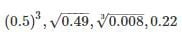Detailed Solution for Quantitative Aptitude - Test 3 - Question 1

Before comparing 1st we will try to bring the given numbers to their simplest form.
(0.5)3 = 0.125
√0.49 = √ (0.7)2 = 0.7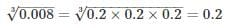0.22
Now, comparing the 4 numbers we can easily say, 1stone is the smallest.
∴ The smallest number is (0.5)3 = 0.125

Quantitative Aptitude - Test 3 - Question 2

###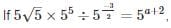then a is equal to

Detailed Solution for Quantitative Aptitude - Test 3 - Question 2

Before we solve this completely, we simplify the LHS using BODMAS rule, and a few rules from indices.
First, we express every quantity in terms of powers of 5.
Hence,
5√5 = 53/2
Hence, we have
53/2 × 5÷ 5-3/2 = 5a+2
Now here, we use BODMAS rule. It is described as follows:
B – Brackets
O – Of
D – Division
M – Multiplication
S – Subtraction
The above is the order in which the operations are to be solved. We observe that there are no brackets or “Of” in the given expression. Hence, we go ahead with division.
5÷ 5-3/2 = 55+3/2 = 513/2
Now, we have multiplication
⇒ 53/2 × 513/2 = 516/2 = 58
Equating the above with RHS,
5= 5a+2
⇒ 8 = a + 2
⇒ a = 6

Quantitative Aptitude - Test 3 - Question 3

### The number of students in a class is increased by 20% and the number now becomes 66. Initially the number was

Detailed Solution for Quantitative Aptitude - Test 3 - Question 3

Let the initial number of students be ‘n’.
Given, number of students in a class is increased by 20% and the number now becomes 66
n + 20% of n = 66
⇒ 1.2n = 66
⇒ n = 55

Quantitative Aptitude - Test 3 - Question 4

The price of a jewel varies as the square of its weight. Two jewels A and B whose weights are in the ratio of 3 and 5 are combined and made a new jewel C. What is the ratio of price of C to the combined price of A and B?

Detailed Solution for Quantitative Aptitude - Test 3 - Question 4

Suppose the weight of the jewel is W and its price is P then according to the question
P ∝ W2,
⇒ P = kW2    where K is the constant.
The ratio between the weights of A and B is 3 : 5. So let’s assume the weight of A is 3x and weight of B is 5x.
So, Price of A = k(3x)2 = 9kx2
And price of B = k(5x)2 = 25kx2
Now, weight of C = weight of A + weight of B = 3x + 5x = 8x
So, price of C = k(8x)2 = 64kx2
Combined price of A and B = 9kx2 + 25kx= 34kx2
So the required ratio = 64kx2 : 34kx2 = 32 : 17.

Quantitative Aptitude - Test 3 - Question 5

4 litres of a 40% solution of spirit in water is mixed with 6 litres of a 50% solution of spirit in water. What is the strength of spirit in the resulting mixture?

Detailed Solution for Quantitative Aptitude - Test 3 - Question 5

Quantity of spirit in 4 litres solution with 40% concentration = (40/100) × 4 = 1.6 litres
Quantity of spirit in 6 litres solution with 50% concentration = (50/100) × 6 = 3.0 litres
Thus, amount of spirit in resulting mixture = 1.6 + 3.0 = 4.6 litres
Total amount of resulting mixture = 4 + 6 = 10 litres
Strength of spirit in resulting mixture = (4.6/10) × 100 = 46%

Quantitative Aptitude - Test 3 - Question 6

If LCM of two numbers is thrice the larger number and difference between the smaller number and the HCF is 14. Then, find out the smaller number of the two numbers.

Detailed Solution for Quantitative Aptitude - Test 3 - Question 6

Solution: Let the larger number be x and the smaller number be y.
It is given that LCM is thrice of the larger number,
Therefore, LCM = 3x      ----(i)
It is also given that difference between the smaller number and the HCF is 14,
Therefore, y – HCF = 14
HCM = y – 14      ----(ii)
We know that product of two numbers = LCM × HCF
Therefore, x × y = 3x × (y – 14)      (from (i) and (ii))
y = 3(y – 14)
y = 3y – 42
2y = 42
y = 21
Hence, the smaller number is 21.

Quantitative Aptitude - Test 3 - Question 7

The ratio of ages of A and B is 11 : 13 respectively. After 7 years the ratio of their ages will be 20 : 23. What is the difference between their ages?

Detailed Solution for Quantitative Aptitude - Test 3 - Question 7

Let the ages of A and B be 11x and 13x respectively. Then,
According to the question, after 7 years the ratio of their ages will be 20 : 23.
⇒ (11x + 7)/(13x + 7) = 20/23
⇒ 260x + 140 = 253x + 161
⇒ 260x – 253x = 161 – 140
⇒ 7x = 21
⇒ x = 3
∴ The difference between their ages = 13x – 11x = 2x = 2 × 3 = 6 years
Hence, the required difference between the ages of A and B is 6 years.

Quantitative Aptitude - Test 3 - Question 8

The value of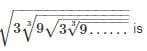Detailed Solution for Quantitative Aptitude - Test 3 - Question 8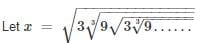On Squaring we get,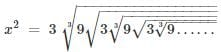On Cubing we get,
x= 27 × 9x
⇒ x5 = 243
⇒ x = 3

Quantitative Aptitude - Test 3 - Question 9

By selling cloth at Rs. 9 per metre, a shopkeeper loses 10%. Find the rate at which it should be sold so as to earn profit of 15%​

Detailed Solution for Quantitative Aptitude - Test 3 - Question 9

Let the CP of 1 m cloth = Rs x
Loss% = 10%
SP of 1 m cloth = Rs 9
Therefore,
Loss = x – 9
10 = ((x – 9)/x × 100%
x – 9 = x/10
x = 10
now CP of 1 m cloth = Rs 10
let the selling price be Rs y
profit% = 15% = ((y – 10)/10 × 100%)
3 = 2(y – 10)
2y – 20 = 3
y = 23/2
y = 11.5
SP of 1 m cloth = Rs. 11.50

Quantitative Aptitude - Test 3 - Question 10

Rs. 3,000 is divided among A, B and C, so that A receives 1/3 as much as B and C together receive and B receives 2/3 as much as A and C together receive. Then share of C is

Detailed Solution for Quantitative Aptitude - Test 3 - Question 10

Given, 3,000 is divided among A, B and C, so that A receives 1/3 as much as B and C together receive and B receives 2/3 as much as A and C together receive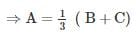⇒ 3A = B + C……….(1)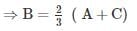⇒ 3B = 2A + 2C…………(2)
⇒ Putting the value of C from (1) into (2), we get,
⇒ 3B = 2 A + 2 (3A – B)
⇒ 8A = 5B …………….(3)
⇒ Similarly, we can solve and will get relation between B and C.
⇒ 7B = 8C …………….. (4)
⇒ Multiplying (3) with 7 and (4) with 5, we get,
⇒ 56A = 35B = 40C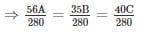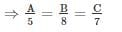⇒ A : B : C = 5 : 8: 7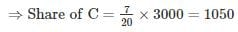Quantitative Aptitude - Test 3 - Question 11

At some rate per annum the amount with compound interest on Rs. 1600 for 2 years is Rs. 1730.56. The rate of interest per annum is:

Detailed Solution for Quantitative Aptitude - Test 3 - Question 11

We know that,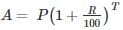Where, A = Final amount, P = Principal amount, R = % rate of interest, T = time period in years
Here, P = Rs. 1600, T = 2 years, A = 1730.56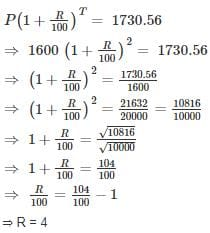Thus, rate of R% per annum is 4%

Quantitative Aptitude - Test 3 - Question 12

I walk a certain distance and ride back taking a total time of 37 minutes. I could walk both ways in 55 minutes. How long would it take me to ride both ways?

Detailed Solution for Quantitative Aptitude - Test 3 - Question 12

Speed = distance/time
Let the distance be x km. Then,
(Time taken to walk x km) + (Time taken to ride x km) = 37 min.
⇒ (Time taken to walk 2x km) + (Time taken to ride 2x km) = 74 min.
Given, time taken to walk 2x km = 55 min.
Time taken to ride 2x km = (74 - 55) min = 19 min

Quantitative Aptitude - Test 3 - Question 13

Two trains start at the same time from two point P and Q and proceed towards each other at the speed of 75 km/hr and 100 km/hr respectively. When they meet, it is found that first train has travelled 125 km less than the second train. Find the distance between point P and Q.

Detailed Solution for Quantitative Aptitude - Test 3 - Question 13

Let the distance travelled by first train till the point they meet be X km. Since the second train travelled 125 Km more than the first one, thus
Distance travelled by second train when they meet = X + 125
We know that, Time = Distance/Speed
We know that both trains travelled for the same time till they meet. Hence,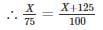⇒ 100X = 75X + 9375
⇒ X = 375 Km
∴ X + 125 = 500 Km
Hence the distance between the points P and Q = Distance travelled by train A & B
= 375 + 500
= 875 Km

Quantitative Aptitude - Test 3 - Question 14

Aman and Akbar together can do a work in 18 days. Both of them began to work. After 4 days Akbar fell ill, Aman completed the remaining work in 42 days. In how many days can Aman complete the whole work if he did it alone?

Detailed Solution for Quantitative Aptitude - Test 3 - Question 14

Let the total work be W units.
Amount of work done by Aman in one day = Am units
Amount of work done by Akbar in one day = Ak units
W = (Am + Ak) 18
Now this piece of work has been started, but both worked for only 4 days, after which Aman took over,
⇒ W = (Am + Ak) 4 + (Am) 42
⇒ (Am + Ak) 18 = (Am + Ak) 4 + (Am)42
⇒ (Am + Ak) 14 = (Am) 42
⇒ Am + Ak = 3 Am
⇒ Ak = 2 Am
Substituting the value of Ak in the very first equation, we have
W = (Am + 2 Am)18
⇒ W = 54 Am
Hence, Aman alone would have taken 54 days to complete the work.

Quantitative Aptitude - Test 3 - Question 15

The sides of a right-angled triangle forming right angle are in the ratio 5 : 12. If the area of the triangle is 270 cm2, then the length of the hypotenuse is

Detailed Solution for Quantitative Aptitude - Test 3 - Question 15

Given data-
Sides of a right-angled triangle forming right angle are in the ratio 5 : 12
Let sides of triangle forming right angle be 5x & 12x respectively, where x is constant of proportionality.
Area of the triangle = ½ × base × height = ½ × 5x × 12x = 30x2
30x2 = 270
⇒ x2 = 9
⇒ x = 3
∴ sides of triangle forming right angle are-
5x = 5 × 3 = 15 cm
& 12x = 12 × 3 = 36 cm
Using Pythagoras theorem
hypotenuse2 = 152 + 362
⇒ hypotenuse2 = 225 + 1296
⇒ hypotenuse2 = 1521
∴ Hypotenuse = √1521 = 39 cm

Quantitative Aptitude - Test 3 - Question 16

Compute the ratio of the volumes of two cylinders if the ratio of radii of the bases is 9 : 4 and the ratio of their heights is 3 : 7

Detailed Solution for Quantitative Aptitude - Test 3 - Question 16

Volume of a cylinder = πr2 × h
Hence the ratio of their volumes
= (πr12 × h1)/(πr22 × h2)
= (92 × 3)/(42 × 7)
= 243/112

Quantitative Aptitude - Test 3 - Question 17

AB and CD are two parallel chords on the opposite sides of the centre of the circle. If AB = 18 cm, CD = 24 cm and the radius of the circle is 15 cm, the distance between the chords is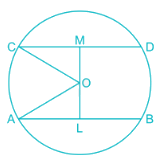Detailed Solution for Quantitative Aptitude - Test 3 - Question 17

Perpendiculars drawn from the centre on the chord bisects them.
Given, AB and CD are two parallel chords on the opposite sides of the centre of the circle.
AB = 18 cm, CD = 24 cm and the radius of the circle is 15 cm
From the figure,
OM and OL are perpendiculars, OC and OA are radius
∴ CM = CD/2 = 12 cm and AL = AB/2 = 9 cm
By Pythagoras theorem:
OC2 = OM2 + MC2
⇒ OM2 = 152 – 122 = 81
⇒ OM = 9 cm
Similarly,
OA2 = OL2 + AL2
⇒ OL2 = 152 – 92 = 144
⇒ OL = 12 cm
Distance between the two chords = OL + OM
⇒ Distance between the two chord = 12 + 9 cm = 21 cm

Quantitative Aptitude - Test 3 - Question 18

Two circles intersect at A and B. P is a point on produced BA. PT and PQ are tangents to the circles. The relation of PT and PQ is

Detailed Solution for Quantitative Aptitude - Test 3 - Question 18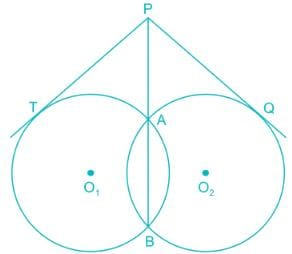We know that,
Property- If MNO is a secant to a circle intersecting the circle at M and N and OT is a tangent segment, then
OM × ON = OT2
From the diagram,
PAB is a secant to a circle with center O1 intersecting the circle at A and B and PT is a tangent segment,
∴ PA × PB = PT2  ……. (1)
Again, PAB is a secant to a circle with center O2 intersecting the circle at A and B and PQ is a tangent segment,
∴ PA × PB = PQ2  ……. (2)
From (1) and (2)
PT2 = PQ2
⇒ PT = PQ

Quantitative Aptitude - Test 3 - Question 19

If XYZ is an equilateral triangle and S is a point on YZ such that XS ⊥ YZ, then

Detailed Solution for Quantitative Aptitude - Test 3 - Question 19

As we know, in an equilateral triangle all sides are equal.
∴ XY = YZ = ZX
Let XY = 2a units
∴ YZ = ZX = 2a units
And XS is perpendicular bisector of side YZ
∴ YS = SZ = a units
Thus, XY: YS = 2: 1

Quantitative Aptitude - Test 3 - Question 20

ABCD is a cyclic trapezium whose sides AD and BC are parallel to each other. If ∠ABC = 72°, then the measure of the ∠BCD is

Detailed Solution for Quantitative Aptitude - Test 3 - Question 20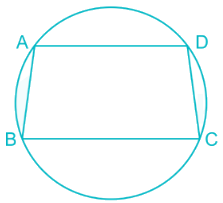As stated in above figure ABCD is a cyclic trapezium with Line AD and Line BC parallel to each other.
As per the properties of cyclic quadrilateral,
Also, Properties of parallel lines
∠BCD = 180° - ∠ADC = 180° - 108° = 72°
∴ ∠ABC = ∠BCD
⇒ ∠BCD= 72°

Quantitative Aptitude - Test 3 - Question 21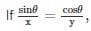sinθx=cosθy,then sinθ + cos θ is equal to

Detailed Solution for Quantitative Aptitude - Test 3 - Question 21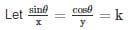∴ sinθ = kx and cosθ = ky
sin2θ + cos2θ = 1
⇒ k2x2 + k2y2 = 1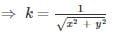sinθ + cos θ = kx + ky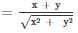Quantitative Aptitude - Test 3 - Question 22

The angle of elevation of the top a tower form the base of a building is 60° and the angle of elevation of the top of the building from the base of the tower is 30°. If the height of tower is 125 m, find the height of the building.

Detailed Solution for Quantitative Aptitude - Test 3 - Question 22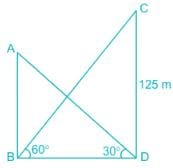Consider AB as height of building and CD be as height of tower
AB=?
From trigonometric formulas,
Tan θ = perpendicular/base
∴ in ΔBCD
tan 60° = CD/BD
⇒ √3 = CD/BD
⇒ 125/√ 3 = BD
Also, in ΔABD
tan 30° = AB/BD
⇒ 125/√ (3 × 3) = AB
⇒ AB = 125/3 = 41.66m

Quantitative Aptitude - Test 3 - Question 23

The value of 2cos220° + 3cos270° + sin270° is

Detailed Solution for Quantitative Aptitude - Test 3 - Question 23

2cos220° + 3cos270° + sin270°
= 2cos220° + 2cos270° + cos270° + sin270°
= 2cos220° + 2cos(90 – 20)° + 1
= 2cos220° + 2sin220° + 1
= 2 + 1 = 3

Quantitative Aptitude - Test 3 - Question 24

5 years ago the average age of a family of 3 members was 19 years. A baby having been born, the average age of the family remains the same today. The age of the baby after 8 years would be

Detailed Solution for Quantitative Aptitude - Test 3 - Question 24

According to the given information,
5 years ago the average age of a family of 3 members was 19 years.
(x – 15)/3 = 19
⇒ x = 72
A baby having been born, the average age of the family remains the same i.e. 19 years
Let the present age of baby be ‘b’ years
⇒ (72+b)/4 = 19
⇒ b = 4
The age of the baby after 8 years would be 4 + 8 = 12 years

Quantitative Aptitude - Test 3 - Question 25

The sum of all odd numbers between 11 and 61 is

Detailed Solution for Quantitative Aptitude - Test 3 - Question 25

Using Sum of Arithmetic Progression
Total odd numbers between 11 and 61, n = 24
Sum = n/2[a + l] = 24/2[13 + 59] (a = first term, l = last term)
= 24/2 × 72
= 864

## SSC CGL Tier 1 Mock Test Series

244 docs|118 tests
Information about Quantitative Aptitude - Test 3 Page
In this test you can find the Exam questions for Quantitative Aptitude - Test 3 solved & explained in the simplest way possible. Besides giving Questions and answers for Quantitative Aptitude - Test 3, EduRev gives you an ample number of Online tests for practice

## SSC CGL Tier 1 Mock Test Series

244 docs|118 tests